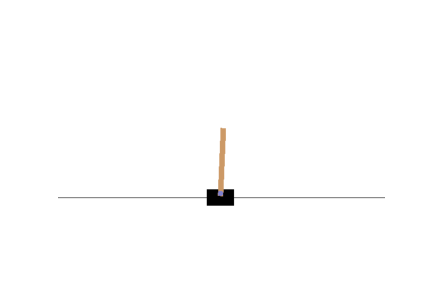# Game with untrained neural network

• Now that we have built the neural network, we shall just see how the neural network - with absolutely no training but with the randomly initialized weights - performs in determining the action to take for the next step of the agent.

• Let's write a small function that will run the model to play one episode, and return the frames so we can display an animation:

INSTRUCTIONS
• Set the `env` seed:

``````env.seed(42)
``````
• Let's code the policy for this untrained network. As discussed above, we shall randomly choose the threshold for deciding on the action(if it should be left, or right). We shall code the same here:

``````def basic_policy_untrained(obs):
left_proba = model.predict(obs.reshape(1, -1))
action = int(np.random.rand() > left_proba)
return action
``````
• Let us run this for 50 episodes and 200 steps. we shall call the `basic_policy_untrained` for each step of each episode:

``````totals = []
for episode in range(50):
episode_rewards = 0
obs = env.reset()
for step in range(200):
action = basic_policy_untrained(obs)
obs, reward, done, info = env.step(action)
episode_rewards += reward
if done:
break
totals.append(episode_rewards)

np.mean(totals), np.std(totals), np.min(totals), np.max(totals)
``````

We know that this occurs by random, it performs pretty bad! Let us see the visual of its performance:It is pretty clear that the cartpole is quite unstable and wobbly. Let us now head towards training the neural network with our basic policy and then see its visual in the next section.

No hints are availble for this assesment

Answer is not availble for this assesment

Note - Having trouble with the assessment engine? Follow the steps listed here